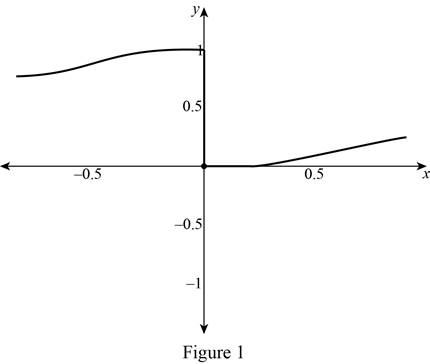# To estimate: The value of lim x → 0 − 1 1 + e 1 x from the graph of f ( x ) = 1 1 + e 1 x .### Single Variable Calculus: Concepts...

4th Edition
James Stewart
Publisher: Cengage Learning
ISBN: 9781337687805### Single Variable Calculus: Concepts...

4th Edition
James Stewart
Publisher: Cengage Learning
ISBN: 9781337687805

#### Solutions

Chapter 2.2, Problem 9E

(a)

To determine

## To estimate: The value of limx→0−11+e1x from the graph of f(x)=11+e1x.

Expert Solution

The value of limx011+e1x=1.

### Explanation of Solution

Using the graphing calculator, the graph of the function f(x)=11+e1x is drawn and shown below in Figure 1.From Figure 1, it is observed that the graph approaches y=1 as x approaches 0 from the left side.

Thus, it is concluded that limx011+e1x=1.

(b)

To determine

### To estimate: The value of limx→0+11+e1x from the graph of f(x)=11+e1x.

Expert Solution

The value of limx0+11+e1x=0.

### Explanation of Solution

From Figure 1, it is observed that the graph approaches y=0 as x approaches 0 from the right side.

Thus, it is concluded that limx0+11+e1x=0.

(c)

To determine

### To estimate: The value of limx→011+e1x from the graph of f(x)=11+e1x.

Expert Solution

The value of limx011+e1x does not exist.

### Explanation of Solution

From Figure 1, it is observed that the graph does not approach any value when x equals exactly 0.

Also, notice the left hand limit and the right limit of limx011+e1x obtained from part (a) and part (b) are not the same.

That is limx011+e1x=1 and limx0+11+e1x=0.

Thus, it can be concluded that limx011+e1x does not exist.

### Have a homework question?

Subscribe to bartleby learn! Ask subject matter experts 30 homework questions each month. Plus, you’ll have access to millions of step-by-step textbook answers!